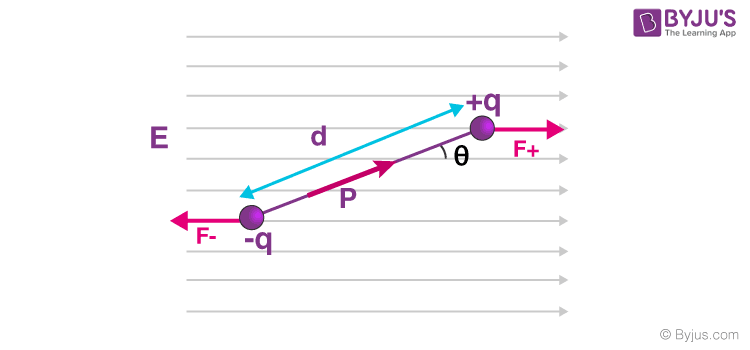# Torque on an Electric Dipole in an Uniform Electric Field

Let’s review our knowledge of torque and electric dipole to better comprehend the characteristics of the torque acting on an electric dipole in a uniform electric field.

### Torque:

• Torque is a measure of the force that can cause an object to rotate about an axis.
• Torque is a vector quantity
• The following formula is used to determine the torque vector’s magnitude:
• $$\begin{array}{l}τ = F \times r\end{array}$$
• $$\begin{array}{l}τ = F \times r \times Sinθ\end{array}$$
• Where,
• r = Position vector (a vector from the point about which the torque is being measured to the point where the force is applied)
• F = Force vector
• θ = Angle between the force vector and the lever arm vector.

### Electric Dipole:

Electric Dipole: Pair of opposite electric charges with equal magnitudes that are lined apart by “d”.
Electric Dipole Moment: The product of the magnitude of these charges (q) and their separation from each other (d).
Direction: From the negative charge to the positive charge.
Formula: p = q x d
Unit: Cm
Quantity: Vector

#### Recommended Video:## Derivation of Torque on an Electric DipoleNow suppose a dipole whose charges “+ q” and “- q” form a dipole because they are separated by “d”. If we place a dipole in a uniform electric field of strength “E”, then the angle between the Electric field & Dipole moment is “θ”.

$$\begin{array}{l}\overrightarrow{F_+} = +q\overrightarrow{E}\end{array}$$
$$\begin{array}{l}\overrightarrow{F_-} = -q\overrightarrow{E}\end{array}$$

The components of force perpendicular to the dipole are:

$$\begin{array}{l}F_{+}^⊥ = +qE~sinθ\end{array}$$
$$\begin{array}{l}F_{-}^⊥ = -qE~sinθ\end{array}$$

Since the force magnitudes are equal and are separated by a distance d, the torque on the dipole is given by:
Torque (τ) = Force × distance seperating forces

$$\begin{array}{l}τ = d~ qE ~sin~θ\end{array}$$

Since dipole moment is given by

$$\begin{array}{l}p = qd\end{array}$$

As can be seen from the equation above, the torque is the cross product of the dipole moment and electric field and the direction of the dipole moment is from the negative to the positive charge. If the direction of the electric field is positive, then the direction of the torque is clockwise (Negative).

$$\begin{array}{l}τ = -pEsinθ\end{array}$$
Or,
$$\begin{array}{l}\overrightarrow{τ} = \overrightarrow{p}~×~\overrightarrow{E}\end{array}$$
$$\begin{array}{l}|\overrightarrow{τ}| = |\overrightarrow{p}~×~\overrightarrow{E}| = pEsinθ\end{array}$$

## Summary

1. Torque is the measure of force that drives an object to revolve around an axis.
2. An electric dipole is a pair of opposite electric charges that are separated from one another by a distance “d”.
3. The torque on the dipole is determined if
• Force magnitudes are equal: “F”
• Separated by a distance: “d”
• Torque (τ) = Force × distance separating forces
4. The torque on an electric dipole in a uniform electric field is given by the equation
• $$\begin{array}{l}\begin{vmatrix} \tau \end{vmatrix} = \begin{vmatrix} \overrightarrow{p} \times \overrightarrow{E}\end{vmatrix} = pE\, sin\theta\end{array}$$

## Frequently Asked Questions – FAQs

Q1

### Describe torque.

Torque is the cross multiplication of force vector and Position vector (a vector from the point about which the torque is being measured to the point where the force is applied).
Q2

### What is the formula of torque?

τ = F r Sinθ
Where,
r = Position vector (a vector from the point about which the torque is being measured to the point where the force is applied)
F = Force vector
θ = Angle between the force vector and the lever arm vector.
Q3

### Can you define “Electric Dipole”?

When the same magnitude of opposite charge is lined by a small distance, “d” is known as “Electric Dipole”.
Q4

### Can you explain “Electric Dipole Moment”?

It is the product of the magnitude of pair of charges (q) and the distance between them (d).
p = qd
The direction of the Electric Dipole moment is from negative charge to positive charge.
Q5

### Describe the electric field?

It is defined as Electric force per unit charge.
E = F/q
SI Unit: N/C
Physical Quantity: Vector
An electric field is a physical field that envelopes electrically activated particles.
Test Your Knowledge On Torque On Dipole!